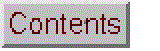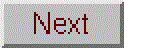# Comparison of analytical calibration methods

This is a set of spreadsheets that perform simulations of widely-used analytical calibration methods. Each spreadsheet simulates an "unknown" sample solution whose concentration is to be measured by an analytical instrument that generates a signal related to concentration. You can specify the "true" concentration of the unknown in the sample solution, then the spreadsheet simulates a measurement of it, using the specified calibration method to establish a calibration based on one or more standard solutions and to convert instrument readings into concentration (including typical experimental errors), reports the calculated result, and compares it to the true value that you specified. To be more realistic, these simulations include typical systematic and random errors in both signal and in volumetric measurements. They demonstrate how non-linearity, interferences, and random errors combine to influence the final result and and they allow you to optimize precision and accuracy of the measurement. All of these spreadsheets are now available in both Excel and OpenOffice Calc versions.

Screen shots and Download links:

 Single external standard Download in Excel or Calc format Two-standard bracket method Download in Excel or Calc format Calibration curve method with linear fit Download in Excel or Calc format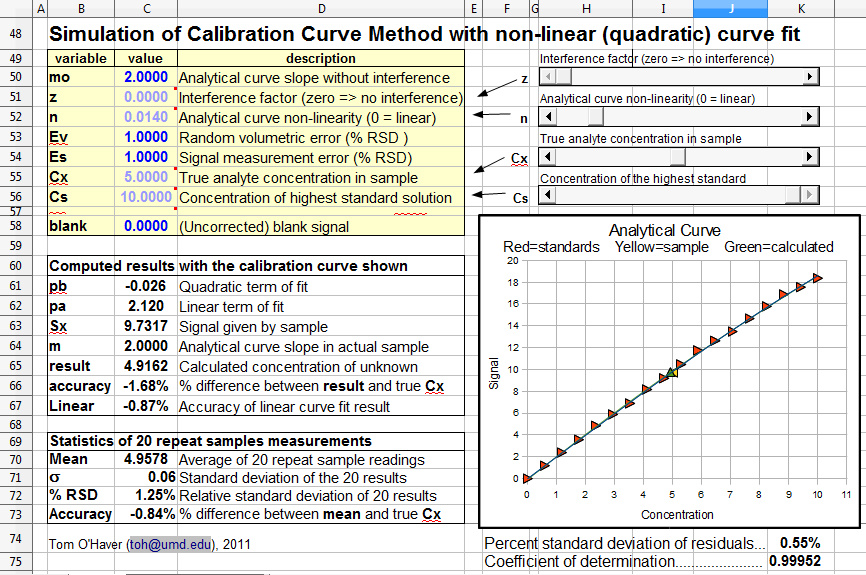Calibration curve method with non-linear fit. Download in Excel or Calc format.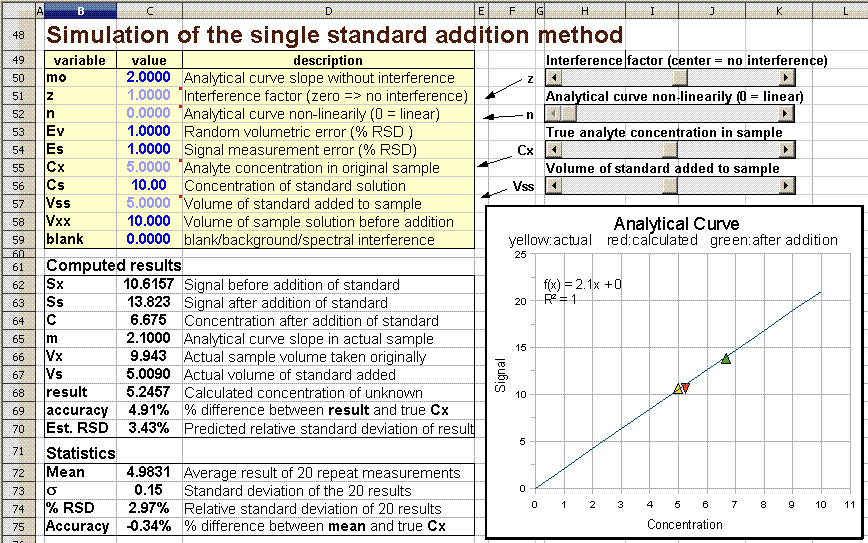Single standard addition method Download in Excel or Calc format Multiple standard addition method Download in Excel or Calc format

Excel versions require a reasonably recent version of Excel. Calc versions require the OpenOffice program, which is a free download from OpenOffice.org for either PCs or Macs. The two versions are mathematically equivalent but slightly different cosmetically.

Related spreadsheets:
Worksheets for linear and non-linear calibration curves for your own data
Comparison of Calibration Curve Fitting Methods in Absorption Spectroscopy
Multiwavelength Spectrophotometric Analysis by Classical Least Squares
Instrumental Deviation from Beer's Law
Calcium Ion Selective Electrode Simulation (Application of single standard addition method)

[Return to Index]

Background.
In analytical chemistry, the accurate quantitative measurement of the composition of samples, for example by various types of spectroscopy, usually requires that the method be calibrated using standard samples of known composition. This is most commonly, but not necessarily, done with solution samples and standards dissolved in a suitable solvent, because of the ease of preparing and diluting accurate and homogeneous mixtures of samples and standards in solution form. (Note: calibration methods may be contrasted to "absolute analytical methods", in which the concentrations of samples are calculated with the aid of previously-measured fundamental data rather than from standards that are measured along with the samples. Absolute methods are occasionally performed when preparing standard samples is difficult or impossible, especially in the measurement of atmospheric gases in situ using laser spectroscopy).

Calibration errors. Calibration procedures are subject to error caused by several complications:

a. Analytical curve non-linearity. The analytical curve is a plot of the signal from the instrument vs the concentration of  the analyte (the chemical species whose concentration is sought). This is closely related to the calibration curve, which is a plot of the signal from the instrument vs the concentration of the standard solutions. In the absence of interferences, the points of the calibration curve should fall along the analytical curve. If the analytical curve is linear, calibration procedures are much simpler, both mathematically and procedurally. If the relationship in non-linear, a series of standard solution must be prepared and measured to establish the shape of the curve, which is time-consuming as well as requiring the use of larger amounts of standard materials (which can be expensive and will eventually require safe disposal). Commonly, calibration curves are observed to be approximately linear over a certain concentration range, but non-linear above that range. In some well-defined cases, the shape of the analytical curve can be predicted, for example in absorption and in fluorescence spectrophotometry.

b. Additive interferences. Ideally, samples and standards should give a zero reading when the analyte concentration is zero. Commonly, the instrument readout is zeroed when a "blank" is measured (a "blank" is a solution containing zero concentration of analyte in the same solvent and containing vessel). But in many cases this is not enough, because some other unknown chemical components that are present in the samples (but not in the standards) are contributing their own signals to the total signal measured. Unless it it possible to resolve (separate) the signal generated by these components from that of the analyte, the signal measured in that case will be higher than it should be, leading to an error in the analysis. This is called an "additive interference", because the signal from the interfering components adds to that from the analyte. So even if the analyte's concentration is zero, you still get a signal from the sample. In spectroscopy, this is often called a "spectral interference".

c. Multiplicative interferences. Ideally, a given concentration of analyte will give the same signal reading in the sample as in the standards (in other words, the slope of the analytical curve is the same in the samples and standards). But sometimes there are conditions or components present in the samples (but not in the standards) that make the analyte's signal stronger or weaker that it is in the standards; it might be a difference in temperature, pH, ionic strength, density, viscosity, surface tension, or a specific chemical component that reacts with or binds with the analyte. This is called an "multiplicative interference", because the analyte's signal is in effect multiplied by some unknown factor. This is distinct from an additive interference, because with a multiplicative interference, you still get a zero signal when the analyte's concentration is zero.

d. Random errors (uncertainty) and the propagation of random errors. In addition to the systematic errors considered above, analytical methods are also subject to random errors (imperfect repeatability) due to several sources. For example, the preparation of analyte and standard solutions is subject to random errors in volumetric measurements, and the instrument's reading itself is subject to random variability due to electronic noise generated by electronic amplifiers and detectors, instability of light sources, and photon noise, especially when the concentration of the analyte is very low. Proper instrument design and careful technique can reduce, but never completely eliminate, such errors.

The size of random errors are usually described by the standard deviation, s, defined as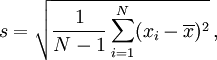where N is the number of data points, xi are the individual points, and x is the mean (average) of all the x's. Spreadsheets and most programming languages have a built-in function for standard deviation. The relative standard deviation, given by s/x, is often also reported, as a percentage.

e. Calculating the propagation of random errors. The way that random errors combine and influence the final precision of the measurement is called propagation of error. When you compute some quantity that is based on two or more measurements, you need to be able to figure out how reproducible the calculated quantity will be when the input variables are subject to random variability. If you know the standard deviation of each of those input measurements, you can calculate the expected standard deviation of the calculated quantity in two different ways:

1. Do the math. By using the rules for mathematical error propagation. In principle the propagation of errors of the entire calibration method can be described by closed-form algebraic formalism by breaking down the equation into a series of simple differences, sums, products, and ratios, and applying the rules for error propagation to each step. However, there are two problems with this approach. If the calculation is complicated, the error propagation can become really complicated and difficult. Secondly, the usual rules for mathematical error propagation assume that the random errors of the various terms of the calculation are not correlated: if they are correlated, the calculations become even more complicated. Correlation between terms occurs in the prediction of error propagation of the bracket and standard addition methods. The bottom line is that it is often difficult to predict the propagation of errors by doing the math.

2. Crunch the numbers. By repeating all the calculations over and over again (obviously using a computer) with random number generators employed to add realistic amounts of random variability ("noise") to the input variables. (This is sometimes called a "Monte Carlo" approach, a reference to the famous gambling casinos in that small country). This is relatively easily set up using spreadsheets, which are well suited to performing laborious repetitive calculations and even have built-in random number and statistical functions. The advantage of this approach over closed-form algebraic formalism is that it can be applied to essentially any arbitrarily-complicated procedure and it automatically takes into account any correlation between variables. The disadvantage is that it is less "elegant" and can not be expressed in a neat formula.

These spreadsheets perform both of these type of calculations, so you can compare them. It's important to understand that even a perfectly accurate calculation of error propagation predicts only the expected standard deviation "on average", for a very large number of repeats. If you were to repeat an actual experiment a few times and compute the standard deviation, you'll often get only a very rough approximation to the theoretical result, perhaps off by 2 or 3-fold. This is a basic problem of statistics in analytical chemistry; the theoretical predictions work well for very large number of repeats, but in analytical chemistry the cost and time of doing even a few repeats is often prohibitive. For this reason it is not worth obsessing about small differences in precision; the statistical uncertainty in measuring the precision of any one method is likely to be greater than the differences between the different methods.

Calibration methods. The methods described below are the most commonly-used analytical calibration methods. Each of these methods, from the simplest to the more complex, is modeled by a separate simulation spreadsheet, which includes all of the above-mentioned systematic errors, plus random errors due to both volumetric measurement and signal measurement. These simulations allow you to investigate how all of these errors combine an propagate to the final result. All of the simulations have a very similar structure and layout, so once you learn how to work the first one, using the others will be relatively straightforward.  The concentration of the unknown sample, Cx, and its predicted standard deviation, is calculated in a different way in each of these calibration methods.

Single external standard (SingleStandard.xlsx or SingleStandardOO.ods):

This is the simplest calibration method, in which the sample and a single separate standard solution are measured. This method assumes that the calibration errors a,b, and c, listed above, are absent.
The concentration of the sample Cx is given by Cs*Sx/Ss, where Cs is the concentration of the standard solution, Ss is the signal given by that standard solution, and Sx is the signal given by the sample solution. The predicted relative standard deviation of Cx is easy to compute in this particular case, if you know the standard deviations of Cs, Sx, and Ss: there are just three variables, all multiplied or divided, so according to the rules for error propagation, the relative standard deviation of Cx is the quadratic sum (square root of the sum of the squares) of the relative standard deviations of Cs, Sx, and Ss, which in this simulation are Ev, Es, and Es, respectively (see cell C68).
Two-standard bracket method (Bracket.xlsx or BracketOO.ods):

In this calibration method, the sample is measured along with two standard solutions that are close in concentration to the sample (typically one lower than and one higher than the sample concentration). This method has the advantage of approximately compensating for non-linearity in the analytical curve, if the two standards are close in concentration to the sample. It's a useful method when you have many samples to analyze that have about the same analyte concentration. However, this method still assumes that calibration error conditions (b) and (c) are absent. A disadvantage of this method is that it requires more time and uses twice the amount of standard material as the single-standard method.
The concentration of the sample Cx is calculated by linear interpolation between two standard solutions and is given by C1s+(C2s-C1s)*(Sx-S1s)/(S2s-S1s), where C1s and C2s are the concentrations of the two standard solutions, S1x and S2s are the signal readings given by the two standards, and Sx is the signal given by the sample solution. The predicted standard deviation of Cx is more complex to compute in this case, but it can be done by breaking down the equation into a series of differences, sums, products, and ratios, and applying the rules for error propagation to each step. (In the spreadsheet "BracketOO.ods", these error propagation calculations are performed in cells C98:F103).
Linear calibration curve method (CalCurve.xlsx or CalCurveOO.ods):

A series of external standard solutions of different concentrations is prepared and measured. A first-order least-squares fit of the data is computed and the resulting equation is used to convert readings of the unknown samples into concentration. An advantage of this method are that the random errors in preparing and reading the standard solutions are averaged over several standards. Moreover, non-linearity in the calibration curve can be detected and avoided (by diluting into the linear range) or compensated (by using non-linear curve fitting methods). An obvious disadvantage of this method is that it requires much more time and uses more standard material than other methods. The calibration data (Cs vs Ss) are fitted with a first-order least-squares fit. (The fit is shown as the straight red line in the graph).

The concentration of the sample Cx is calculated by Cx = (Sx-intercept)/slope, where Sx is the signal given by the sample solution, and "slope" and "intercept" are the results of the least-squares fit. (In this simulation, rather than choosing each standard solution separately, you choose the number of standards (from 2 to 18) and the concentration of the highest one; the other standards are automatically evenly distributed between zero and the specified highest). The predicted standard deviation of Cx is computed from the standard deviations of the slope and intercept given by the curve fitting procedure and the standard deviations of Sx, as shown in cells D111:F119.
Non-linear calibration curve method (CalCurveQuadFit.xlsx or CalCurveQuadFitOO.ods):
A series of external standard solutions is prepared and measured. A non-linear model function is selected that is expected to be a good fit to the calibration data (e.g. a quadratic or cubic function), a least-squares fit of that model to the data is computed, and the resulting non-linear equation is solved for concentration and used to convert readings of the unknown samples into concentration. An advantage of this method is that non-linearity in the calibration curve is compensated at least approximately, depending on the nature and severity of the non-linearity of the calibration curve and the choice of non-linear fitting model equation.  The calibration data (Cs vs Ss) are fitted with a second-order (quadratic) least-squares fit. (The fit is shown as the curved red line in the graph).
The concentration of the sample Cx is calculated by the "Quadratic equation": Cx = (-b+SQRT(b^2-4*a*(c-Sx)))/(2*a) where Sx is the signal given by the sample solution, and a, b, and c are the coefficients from the quadratic least-square fit (quadratic, linear, and intercept, respectively). (In this simulation, the number of standards is fixed at 18; you can choose the concentration of the highest one and the other 17 standards are automatically evenly distributed between zero and the specified highest concentration).
Single addition method (SingleAddition.xlsx or SingleAdditionOO.ods):

In this method, the sample is divided into two portions: one is measured unmodified and the other is "doped" with the addition of a small amount of pure standard and then measured. This method has the advantage of compensating for multiplicative interferences (c, above), but it still assumes that the analytical curve is linear and that additive interferences are absent.
The concentration of the sample is given by (Sx*Vss*Cs)/(Ss*(Vxx+Vss)-Sx*Vxx), where Cs is the concentration of the standard solution, Sx is the signal given by that sample solution by itself, and Ss is the signal given by the sample solution after the addition of standard, and Vxx and Vss are the volumes of the samples and standard solution. The predicted standard deviation of Cx is computed by breaking down the equation into a series of differences, sums, products, and ratios, and applying the rules for error propagation to each step. These error propagation calculations are performed in cells D111:F118.

Multiple Standard addition method (StandardAddition.xlsx or StandardAdditionOO.ods):

A series of aliquots of the sample solution are taken, increasing amounts of standard material are added to each one, and the signals from the resulting mixtures are measured and plotted against the concentration of added standard. If the resulting calibration curve is sufficiently linear, a first-order least-squares fit of the data is computed. The sample concentration is given by the negative of the x-axis intercept (and to the ratio of the y-intercept to the slope). This method has the advantage of compensating for multiplicative interferences. Compared to the single addition method (below), this method reduces the random errors in preparing and reading the standard solutions. Moreover, non-linearity in the calibration curve can be detected and avoided (by diluting into the linear range) or compensated (by using non-linear curve fitting methods). An obvious disadvantage of this method is that it requires much more time and uses more standard material than most other methods.
The concentration of the sample is given by intercept/slope, where "slope" and "intercept" are the results of the first-order least-squares fit of the standard addition calibration curve, shown as the straight red line in the graph. The predicted relative standard deviation of Cx is the quadratic sum (square root of the sum of the squares) of the relative standard deviations of the slope and intercept computed by the curve fitting procedure. These error propagation calculations are performed in cells B82:F87.
When you are using these spreadsheets, you can inspect the equations that perform these calculations by clicking on a calculated cell and looking for the equation that calculates that cell in the rectangular box at the top of the screen. You'll notice that some cells in these spreadsheets have a tiny red squares in their upper right corners; that means they have an attached note, which you can read just by mousing over the cell without clicking.

Brief operating Instructions.

The screen display of each of the simulations have five similar areas:The yellow  table on the top left of the screen are independent variables that you can change. Click on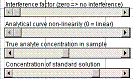the dark blue boldface numbers, type a new value and press the enter key. In the OpenOffice Calc versions, some of these variables can be controlled continuously by the sliders on the top right; in the Excel versions, just type directly into these cells. (The units of concentration in these simulations are normalized to the range of 0 - 10 for convenience in entering and plotting; you can think of them as mmoles/liter, µmoles/liter, grams/liter or any other convenient unit (1 mmole = 0.001 moles; 1 µmole = 10-6 moles). Similarly, the signal units are arbitrary for similar reasons).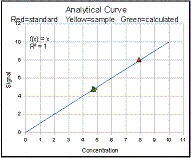The graph on the lower right shows the actual analytical curve (blue line) over the concentration range from 0 to 10 (arbitrary units), with the actual concentration of the unknown sample marked as a yellow triangle. The red triangles are the standards. The green triangle is the calculated concentration of the unknown sample according to that calibration method. In the linear calibration curve and multiple standard addition methods, the red line represents the linear least-squares fit to the calibration curve. The graph responds dynamically as you adjust the variables (e.g. with the sliders).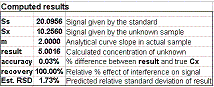The table in the middle left of the screen, labeled "Computed results", are dependent variables that are automatically calculated from the independent variables (don't type in those cells or you will delete the formulae). The most important dependent variable is "result", which is a single simulated experimental measurement of the analyte concentration Cx based on that calibration method.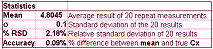The "Statistics" section at the lower left of the screen shows the mean, standard deviation, and % relative standard deviation (%RSD) of 20 simulated repeat calibrations and measurements of the unknown concentration Cx. Here, a repeat calibration means that a complete set of new standards are prepared for each repeat. This gives an idea of the reproducibility if the entire procedure is repeated. The "% RSD" in the table is the relative standard deviation of  20 repeated simulated calibrations; it can be compared to the "Est. RSD" in the Computed results table above, which is the predicted relative standard. based on propagation or error rules.  Both should ideally be the same, but  for a variety of reasons  will usually not agree exactly. The statistics are re-calculated each time an input variable is changed or a slider is moved.

In addition to these user-interface areas, there are "off-screen" areas, below and to the right, that are used by the spreadsheet for graphing, statistics, and error propagation calculations. You don't need to change anything there, but you may inspect those areas if you are interested in the technical aspects of how the simulation spreadsheets work internally.

Assumptions:
1. The only sources of random error are random errors in volume and signal measurement. They apply equally to all solutions and readings of the samples and of the standards. Errors due to interference and blank correction errors apply only to the sample readings and are systematic (constant between measurements).
2. Random errors are expressed as a percentage of the quantity measured (relative error rather than absolute error).
3. Non-linearity of the analytical curve is introduced by a quadratic term whose coefficient is the variable "n" (controlled by the first slider).  (This is not rigorously realistic in the case of the non-linearity in absorption spectroscopy caused by polychromaticity and unabsorbed stray light.  See Instrumental Deviation from Beer's Law for a treatment of non-linearity in that particular case).

Cell definitions and equations (for Bracket method, OpenOffice version):
Inputs:
mo : Analytical curve slope without interference
z : Interference factor (zero -> no interference)
n : Analytical curve non-linearly (0 = linear)
Ev : Random volumetric error (% RSD )
Es : Signal measurement error (% RSD)
Cx : True analyte concentration in sample
C1s : Concentration of standard solution 1
C2s : Concentration of standard solution 2
blank : (Uncorrected) blank signal

Outputs:

Analytical curve slope in actual sample
m = mo+z

Signal given by standard 1
S1s =(mo*C1s-mo*C1s^2*n) * (1+0.01*2.5*Ev*(RAND()-RAND())) * (1+0.01*2.5*Es*(RAND()-RAND()))

Signal given by standard 2
S2s =(mo*C2s-mo*C2s^2*n) * (1+0.01*2.5*Ev*(RAND()-RAND())) * (1+0.01*2.5*Es*(RAND()-RAND()))

Signal given by unknown sample
Sx =(blank+m*Cx-m*Cx^2*n) * (1+0.01*2.5*Ev*(RAND()-RAND())) * (1+0.01*2.5*Es*(RAND()-RAND()))

Measured analyte concentration in sample
result = C1s+(C2s-C1s)*(Sx-S1s)/(S2s-S1s)

Relative percent accuracy
accuracy = (result-Cx)/Cx

Relative % effect of interference on signal
recovery = m/mo

Array calculations for statistics (performed off-screen):
Average: mean = AVERAGE(I99:I118)
Standard deviation: s = STDEV(I99:I118)
Relative standard deviation: RSD = s/mean
Accuracy = (mean-Cx)/Cx

Note: The formulation 2.5*(RAND()-RAND()) seen in the above equations is simply a way of generating random numbers with a "haystack" distribution, a mean of zero and a standard deviation of 1.0, using the RAND() function that by itself gives a uniform distribution between 0 and 1.

Suggested activity: OpenOffice versions.

## Error propagation in analytical calibration methods: Step by Step

[Single standard]  [Bracket Method]  [Calibration curve, linear]  [Calibration curve, non-linear]
[Single Standard Addition]  [Multiple Standard Addition]

### The Single External Standard Method

1. Open SingleStandard.xlxs or SingleStandardOO.ods. This is the simplest calibration method, in which the only two things measured are the unknown sample and a single separate standard solution of known concentration.  The table in the upper left lists the variables that you can change in this simulation.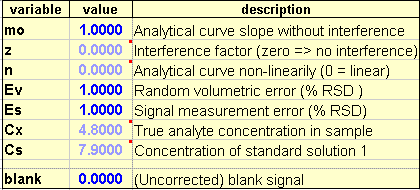The most important one is Cx, which is the true concentration of the sample solution.  (Of course, in the real world, you wouldn't know this beforehand, but in these simulations you can set the true sample concentration as you wish.  The simulation "pretends not to know" the true value and computes the measured sample concentration from the sample and standard signals, just as you would in the real world, then compares that calculated value to the true value to determine the accuracy of the simulated measurement).  The other important variable is Cs, the concentration of the standard solution that you prepare to calibrate the system.  The variable mo controls the slope of the analytical curve, that is, the magnitude of the simulated signals.

The other variables control simulated imperfections and sources of error: z controls multiplicative interferences, blank controls additive interference, n controls the non-linearity of the analytical curve, and Ev and Es control the random errors in volume measurement and signal measures, respectively.  If these variable are set to zero, the simulated measurement should be perfect.

In the OpenOffice Calc versions, some of these variables can be controlled continuously by the sliders on the top right; in the Excel versions, just type directly into these cells in the Value column.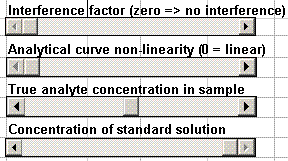The table in the center left lists the quantities that are computed by the simulation.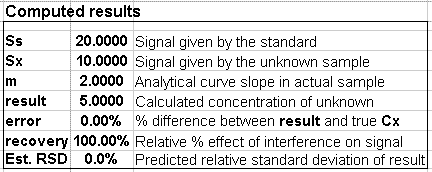The most important of these is the measured concentration of the sample, "result".  In this calibration method, it is given by Cs*Sx/Ss), where Cs is the concentration of the standard solution, Ss is the signal given by that standard solution, and Sx is the signal given by the sample solution.  You can click on the numbers in this table and look at the input line at the top to see the equations that the simulation uses to calculate that number.  The measured signals, Sx and Ss, take into account all the sources of error due interferences, non-linearity, and random errors.  The "Est. RSD" is the estimated relative standard deviation of the result, computed as described above for that calibration method.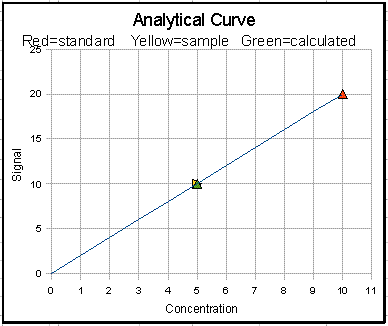The graph on the lower right shows the actual analytical curve (blue line) over the concentration range from 0 to 10 (arbitrary units), with the actual concentration of the unknown sample marked as a yellow triangle. The red triangle represents the standard solution. The green triangle is the calculated concentration of the unknown sample (which should ideally overlay exactly the yellow triangle representing the true sample concentration). The entire graph responds dynamically as you adjust the variables (e.g. with the sliders in the Calc version).

The "Statistics" section at the lower left of the screen shows the result of 20 simulated repeat measurements. The statistics are re-calculated each time an input variable is changed.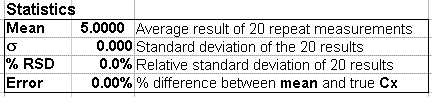Start the experiment with mo=2, blank=0, Ev and Es=0 and set z=0, n=0, Cx=5, and Cs=10. This represents an ideal case - a perfect world with no interferences, no random errors and a perfectly linear analytical curve.  Under these conditions, the sample gives a reading of Sx=10.000 units and the standard gives a reading of Ss=20.000 units. So of course the calibration works perfectly and the "result" equals the true Cx = Cs*Sx/Ss = 10*10/20 = 5.000. The error, percent difference between the true and measured concentrations, is zero. Now vary Cx to other values and you'll see that the results remain perfect for any value of Cx.  Now vary Cs and you'll see that it also have no effect, as long as it is not zero. Even "mo", the slope of the analytical curve, also has no effect as long as it is not zero, because it effect the signals of samples and standards equally.

Now let's make the simulation a little more realistic by introducing some random variability. There are two variables here, the random volumetric error Ev, and the random signal error Es. The random volumetric error refers to the random error in measuring volume or weights when preparing the sample and standard solutions. The magnitude of these errors depends on the technique used and on the solution volumes involved. Then using accurate quantitative glassware (volumetric flasks and pipettes) for volumes in the 10 mL - 1 L range, a volumetric precision of 0.1% is achievable, but a very small volumes below 1 mL  a volumetric precision of 1% is more likely. The signal measurement error refers to the reproducibility of the signal output of the instrument, that is, the signal-to-noise ratio. This varies greatly with the analytical instrument type and the concentration level of the analyte. It may be as good as 0.1% under optimum conditions, but is more likely to be in the 1-10% range, especially at lower concentrations. The signal measurement error, like the random volumetric error, applies to both the sample and the standard.  Both errors are expressed in terms of the relative standard deviation (ratio of the standard deviation to the mean).

For starters, set Ev = 1% and Es = 1%. Now you'll see that the instrument readings Ss and Sx are no longer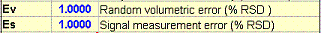exactly 20.000 and 10.000 as before; they are a little off because of the effect of random signal measurement error. If you click on the numbers for Ss and Sx, and look at the entry bar at the top, you'll see the equations for this numbers. Note that they both involve Es because the same instrument is used to measure both Ss and Sx. This causes the calculated sample concentration "result" to be a little off as well. In fact, if you press the `f9` function key at the top of your keyboard, it will cause the spreadsheet to recalculate with different random errors. You can see the Ss and Sx and result jumping around slightly each time you press `f9`. (Also, the little triangles on the graph move ever so slightly). But actually the spreadsheet does this automatically, in the Statistics table.

The
Statistics table, in the bottom left, computes the mean, the standard deviation, and the percent relative standard deviation (% RSD) of 20 repeat measurements (including both signal measurement and volumetric error). Notice that the predicted % RSD of result (in cell C68) is actually larger that the 1% RSD that you set for the random signal measurement error Es. Why? That's because Cs, Ss and Sx are subject to random errors: Cs is subject to random error Ev and Ss and Sx are subject to random error Es. But the errors do not simply add up linearly.

Theoretically, according to the rules for mathematical error propagation,
the % RSD of Cx is predicted to be =SQRT((Es)^2+ (Es)^2+(Ev)^2)/100, if the errors are independent and uncorrelated. If Es = Ev = 1.00, as in this illustration, this works out to about 1.7%.  This is reported as the "Est. RSD". But this is the predicted standard deviation "on average", for a very large number of repeats. Cell C72 gives the actual % RSD of 20 simulated repeat experiments, which should turn out to be somewhere around the Est. RSD, but not exactly because 20 repeats is not really a sufficiently "large" number from a statistical point of view.  But from an analytical laboratory of view, doing 20 repeats of an analytical calibration is a lot of work, time, and expense. Sometimes you can only afford to do 3 or 4 repeats, in which case you'll get an experimental RSD even more approximate, possibly differing from the predicted by a factor of 2 or 3.

This is a basic problem of statistics in analytical chemistry; the theoretical predictions work well for very large number of repeats, but in analytical chemistry the cost and time of doing even a few repeats is often prohibitive).

Now let's introduce a larger random error. Set Es = Ev = 5% and look at the % RSD of the result.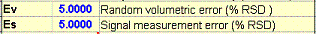It's much larger than before - theoretically 8.7% - because of the extra effect of Ev. But again the actual results bounce around quite a bit as you press f9, in this case mostly between 7 and 11%. What if you use accurate volumetric glassware (which can have an RSD of 0.1%) and a highly precise measurement technique that also gives a 0.1% RSD signal measurement precision)?  Set Es = Ev = 0.1% and see what you get for the % RSD of result.

Now let's make the simulation even more realistic by introducing interferences. Set z to about 0.5, which causes the analytical signal in thesamples to be substantially stronger than that in the standards (this is type of multiplicative interference). Note that this causes the calculated Cx to increase about 25% (as indicated by the Accuracy). Clearly, the single standard method can not compensate for this type of interference. Note: interferences are systematic errors that effect the accuracy but not the precision (% RSD).

Return z to zero and set "blank" = 1. This simulates an additive interference, such as a spectral overlap or background interference. Note that this causes the calculated Cx to be too high (Accuracy is about 10%). Clearly, the single standard method can not compensate for this type of interference either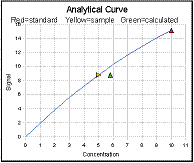Now test the effect of analytical curve linearity. Return "blank" to zero.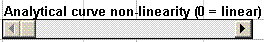Increase n, the "Analytical curve non-linearity" and watch the analytical curve (blue line) in the graph. As n increases, the curve becomes concave down and the accuracy degrades as the curvature increases, as indicated by the fact that the green triangle on the graph (representing the calculated concentration of the unknown sample) is no longer on top of the yellow triangle (representing the true concentration). Clearly, the single standard method depends on having a linear analytical curve. But the problem is that, in the real world, you wouldn't even have a clue that the analytical curve is non-linear if you used only one standard. For that, you'd need to measure more than a single standard.

### The Two-Standard Bracket Method

Open Bracket.xlsx or BracketOO.ods. In this method the sample is measured along with two standard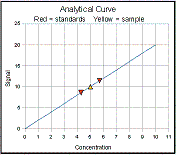solutions that are (ideally) close in concentration to the sample (typically one lower than and one higher than the expected sample concentration). The concentration of the sample Cx is calculated by linear interpolation between the two standards (cell C65)Note in the yellow table that there are two entries for the concentrations of the two standard solutions (called 1 and 2 in the Calc version and a and b in the Excel version). The closer the the two standards are together, the smaller the error due to analytical curve non-linearity.  Of course, this assumes that know the sample concentrations beforehand, at least approximately, in order to be able to make up appropriate bracketing standards.  (For this reason, the two-standard bracket method is mostly used when the approximate range of unknown concentrations is narrow and fairly well known, as in quality control applications.  It is not well suited when there are a large number of samples of widely and unpredictable varying concentrations. (In very careful, high-accuracy work, a preliminary measurement is made based on the single-standard or calibration curve method, then that result is used to prepare two standards of slightly greater and slightly lower concentrations for a second measurement by the bracket method).

Start the experiment with mo=2, blank=0, Ev and Es=0 and set z=0, n=0, Cx=5, and C1s=4,3 and C2s=5.7. In this case (linear calibration curve, zero noise) everything works perfectly.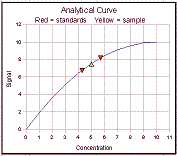Now, increase the non-linearity (n) gradually and watch the shape of theanalytic curve (blue) change. Note that the error (cell 66) stays fairly low, even as the calibration curve becomes noticeably non-linear. Even when n=0.1, the error is less than 1%, compared to a 5% error for the single-standard method with Cs=5.7.  So the the two-standard bracket method is effective in reducing, but not completely eliminating, the non-linearity error.

Try changing Cx just outside the range of the two standards; it still works pretty well as long you don't get too far off.  Cx does not actually have to fall between the two standards, just close to them.

Now set Ev and Es=1.Note that the predicted RSD (based on error-propagation calculations) is greater than the measured RSD in the statistics section. This is caused by the correlation between the terms in the expression for sample concentration; simple error propagation math won't work well in this case. Comparing the measured RSD of this method with that of the previous (single standard) method, you can see that the two-standard bracket method is very slightly less precise, because of the random error in preparing and measuring two standards rather than one, but this hardly matters if the analytical curve suffers from a significant curve non-linearity that the bracket method can compensate for.

### The Calibration Curve Method with Linear Curve Fit

Open CalCurveOO.ods (view Screen Shot). This simulates a calibration curve with 2 to 18 standard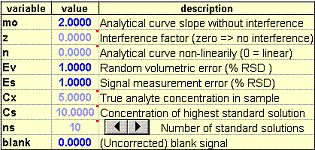solutions and a linear least-squares fit. This is probably the most common calibration method in general use. It is laid out just like the previous simulations, with a few additions. You can choose the number of standards (ns) by typing into cell C57 or by clicking on the arrowheads of the "spinner button" (Calc or Excel version). Cs now controls the concentration of the highest standard solution.  The concentrations of the other standards are spaced out evenly between 0 and Cs.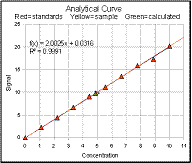The
slope and intercept of the linear least-squares fit to the calibration curve (the red line on the graph) is shown in the computed results section, and the equation of the fit and the R2 value (the "Coefficient of Determination", sometimes called the "Correlation Coefficient") is shown in the upper left of the graph.  The R2 value is one way to estimate the "goodness-of-fit" of the least-squares line to the data; it is 1.000 when the fit is perfect and less than 1.000 when the fit is imperfect.

To start with, set mo=2, blank=0, Ev and Es=0, and set z=0, n=0, Cx=5, and Cs=10. Now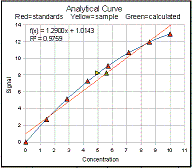increase the non-linearity (variable "n") to introduce a little non-linearity. As the analytic curve becomes more curved, you can clearly see that the linear least-squares fit no longer describes the curve well. Also, you'll see the R2, which is 1.000 for a perfect straight-line, begin to drop gradually, but R2 still reads 0.99 when the curve is already severely non-linear and the error has already begun to degrade seriously (see error in the Statistics table) to about 7% error. Even an R2 value of 0.999 results in an error of 2%. Maybe 2% sounds pretty good, and in some applications that may be adequate, but sometimes analytical methods are called upon to make measurements as accurate as 0.1% or even better. So this tells us that R2 must be expressed to several (3 or 4) decimal places for analytical calibration purposes.

Test this simulation also for interference (variables "z" and "blank"); you'll see that it is no better than the single standard method that that respect.

Set Ev and Es=1 to introduce a small random error.You'll see some small random scatter in the calibration points, with some slightly above and some slightly below the "best fit" line in red, and the R2 value will drop slightly below 1.0. Also the measured Cx ("result") will no longer be exact. In the Statistics section, the entire calibration curve and measurement procedure is repeated 20 times (not just 20 repeat readings of the sample). With the conditions set the same as before (mo=2, blank=0, Ev and Es=1, z=0, n=0, Cx=5, and Cs=10, and ns=2) you'll notice that the %RSD in the statistics section is slightly higher than Es and Ev (around 1.4%). The increase is caused by the variability of the calibration curve.

There is really no way to prepare a perfect calibration curve without random error. But is is possible to reduce the reduce the variability
of the computed slope and intercept of the calibration curve by using more standards, thereby "averaging out" some of the random variability. Try setting ns to 2 and then to 18. Note that the measured Cx ("result") is more more accurate and that the %RSD is also lower (about 1) with the higher number of standards. This is what you get in return for all that extra work of preparing and running a larger number of standards.  Whether it's worth it or not depends on the situation. Compared to the single-standard method, the calibration curve method give a slightly lower %RSD as long as the number of standards is greater than 2, because a calibration based on several standards is better than one based on a single standard. However, the difference is not as much as you might think, because the reading of the unknown signal Sx has the same uncertainty as in the single standard method, and that uncertainty is not decreased by using more standards.

How can we predict how much random error we can expect in the result (Cx), without performing a series of experiments or creating a simulation? The standard way to do this is to perform a propagation of error calculation on the least-squares slope and intercept and on the equation that calculates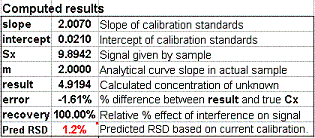the sample concentration: namely Cx = (Sx-intercept)/slope. This is done in the table D110:F119, and the result of this calculation is shown as "Pred RSD" in cell C74. The prediction is based only on a single calibration curve and is good only insofar as that calibration curve is typical of others that might be obtained in repeated trials.  If your random errors happen to be small when you run your calibration curve, you'll get a deceptively good-looking calibration curve, but then your estimates of the random error in the slope and intercept will be too low. If your random errors happen to be large, you'll get a deceptively bad-looking calibration curve, but then your estimates of the random error in the slope and intercept will be too high.  (Here are two examples taken from a set of 20 repeats, one "good" and one "bad", that illustrate this point). Some days it just does not pay to be lucky.

One way to help this situation is to use more standards. Try varying the
number of standards, ns; you will also discover that, if the number of standards is very small, the agreement between the "Predicted % RSD"  and the % RSD of 20 repeat calibrations is very poor. As the number of standards increases, then agreement improves and the actual error decreases. What's the minimum number of standards needed? There is no hard and fast answer to that question; it all depends on the quality of the data and the required quality of results.

These simulated experiments demonstrate two things: first, the predicted RSD (because it is based on a single calibration curve) is extremely unreliable when the number of standards is small, and second, the %RSD of the result improves slightly when the number of standards is increased greatly. We rightly expect that the precision of measurement of concentration should improve if more standards are used, but not so much as you might expect. Looking at the expression for the sample concentration, Cx = (Sx-intercept)/slope, the precision of the slope and intercept are inversely proportional to the square root of number of standards, ns, but the precision of Sx does not depend on the number of standards. For example, if we go from using 4 standards to using 16 standards (4 times as many), the RSD the slope and intercept does decrease by half (the square root of 4), but the RSD of calculated concentrations decreases only from 1.5% to 1.2%. So, using a larger number of standards has some benefits, but it may or may not be "worth it" considering the time and expense of preparing and running more standards.

### The Calibration Curve Method with Non-Linear Curve Fit

Open CalCurveQuadFit.xlsx or CalCurveQuadFitOO.ods (view Screen Shot). This simulates a calibration curve with multiple standards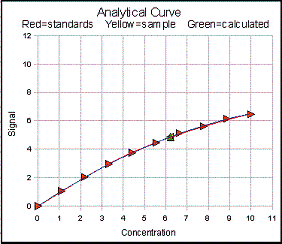solutions and a quadratic least-squares fit.  Set the usual starting conditions: mo=2, blank=0, Ev and Es=0 and set z=0, n=0, Cx=5, and Cs=10. Obviously in this perfect linear case the results are essentially perfect (zero standard deviation, almost perfect accuracy, and R2 = 1.000).Now increase the calibration curve non-linearity, n, to about  n=0.05 and compare the error (in cell C66) with the error of the linear method (in adjacent cell B67). You will find that this method is effective at fitting moderate degrees of non-linearity, and (unlike the bracket method) it does so over the entire range of concentrations (test this by varying the Cx slider). It fails, however, if the analytical curve is too non-linear, especially if it goes to a flat plateau or doubles back on itself. Try increasing n all the way up to 0.1 and note the error is not so low.

Another problem with non-linear fits occurs when there is lots of random error (noise) in the data. Return n to 0.05 (
half-way up) and set Ev and Es=1. With a modest amount of random noise such as this, the quadratic fit works pretty well. Compare the error (in cell C66) with the error of the linear method (in adjacent cell B67). In this case, the non-linearity is the dominant source of inaccuracy.  Note that the relative standard deviation of 20 repeat calibrations (cell C72) is about 2%, a little higher than a linear calibration curve with 10 standards (about 1.5%), but that's hardly a deal-breaker if the error due to non-linearity is greater than that due to random noise.

But now set
Ev and Es=5. Press the f9 key a few times to simulate different calibration curves. Now the plot shows a good bit of discrepancy between the actual analytical curve (blue) and the quadratic fit to the data points (red). The curve fit does its best to fit the data points, even if it has to weave a wavy line through and between the points. With more random error, you can get some truly strange fits in some cases.

The bottom line is that, if you know from previous experience that the true calibration curve is linear, then a linear fit will be better than a non-linear fit, especially if the data are very noisy, because
a non-linear fit will try to "fit the noise", occasionally leading to very great errors.  If the calibration curve is clearly non-linear, and the potential errors due to linear curve-fitting are greater than the random errors due to noise, then a non-linear fit is a good choice. On the other hand, a linear fit may be best with really noisy data, even if the calibration curve is slightly non-linear, because the error caused by a non-linear fit trying to "fit the noise" may exceed the error caused by a linear fits inability to fit the curve.

Reversed-axis fits (Optional):  The application of curve fitting to analytical calibration requires that the fitting equation be solved for concentration as a function of signal in order to be applied to the measurements of unknown samples.  This is trivial in the case of a linear fit, and not so hard for a quadratic fit (requiring the use of the well-known "quadratic equation" found in any algebra textbook), but it becomes more difficult for higher-order polynomial fits. One technique that is sometimes used in these cases is to reverse the x and y axes, that is, to plot concentration on the y axis as a function of signal on the x axis.  This is not really justified statistically, but is is nevertheless sometimes done in practice because it avoids the need to solve the fitting equation.  For example, consider the quadratic fit: in a normal quadratic fit (plotting concentration on the x axis and signal on the y axis as usual), the concentration of unknowns is calculated using the quadratic equation as Cx = (-b+SQRT(b^2-4*a*(c-Sx)))/(2*a), where Sx is the signal given by the unknown sample solution, and a, b, and c are the coefficients from the quadratic least-square fit (quadratic, linear, and intercept terms, respectively).  If the axes are reversed (plotting concentration on the y axis and signal on the x axis), the concentration of unknowns is calculated by the simpler expression Cx = ax^2+Bx+(c-Sx).  The practical difference between these two approaches is demonstrated by the spreadsheet NormalVsReversedQuadFit2.ods (Screen shot), which applies both techniques to the same set of simulated calibration data. This spreadsheet shows that the normal method is in fact slightly better on average, although the difference is slight in most cases, especially if the random errors in signal reading (Es) and in concentration (Ev) are comparable. Admittedly, the reversed-axis approach is really not needed for the quadratic case. It us usually used only for cubic and higher-order fits, where the difficulty of solving the fitting equation is much greater; for example, CalCurveCubicFitOO.ods (Screen shot) applies this technique to a cubic (third-degree) calibration fit, and it could be easily extended to even higher order polynomial fits, even those for which the solution of the fitting equation is actually mathematically impossible. A related spreadsheet (ReversedQuadraticVsCubic.ods, Screen shot) compares the reversed quadratic and reversed cubic fits applied to the same calibration data, showing that there is nothing significant to be gained by going to a cubic fit, at least for the type of non-linearity simulated here.  On the other hand, the cubic fit can be useful in some practical cases where the non-linearity of the analytical curve is not well matched by a quadratic fit; a common example is absorption spectroscopy (see BeersLawCurveFit.html).

Technical note:  All of these these non-linear curve fitting spreadsheets use the LINEST function (common to Excel and OpenOffice Calc). For example, in cell B136 of CalCurveCubicFitOO.ods, the syntax is LINEST(E117:E126;B117:D126;0;0), where E117:E126 are the 10 concentrations of the standards, D117:D126 are the measured absorbances, C117:C126 are the absorbances squared, and B117:B126 are the absorbances cubed. (Important detail: Because this is an array function, rather than a normal function, when you enter this function into the cell you have to press Ctrl-Shift-Enter rather than just Enter). The function returns the first-order coefficient (equivalent to the slope) in cell B136 (the variable named "qa" in the spreadsheet), the second-order coefficient in cell C137 (the variable "qb"), and the third-order coefficient in cell D137 (the variable "qc"). The constant term is zero. These coefficients are then used to compute the concentrations C of unknown samples from their measured absorbance A: C = qa*A+qb*A2+qc*A3.  In the Statistics section, this entire cubic calibration procedure is repeated 20 times, in the 20 bordered blocks of cells that extend to the right between rows 115 and 140 out to column DP, and the results for each repeat are collected in the Results table in column J.

### The Single Standard Addition Method

Open (SingleAddition.xlsx or SingleStandardOO.ods (view Screen Shot).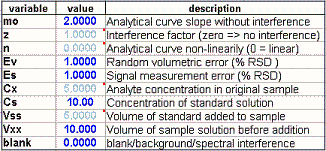In this method, the sample is divided into two portions: one is measured unmodified and the other is "doped" with the addition of a known small volume of pure standard of known concentration and then the mixture is measured. This is similar to the single standard method, in that only the sample and a single standard are measured, but the difference is that in this case the standard solution is in the same matrix as the sample, so it is effected by the same multiplicative interference, no matter what the origin of that interference might be.

The downside of this method is that each separate sample requires the preparation of its own standard, whereas in the other methods one standard (or one set of standards) can be used to analyze a whole series of different samples. Also, the calculations must compensate for the fact that the concentration of the standard solution now contains an unknown contribution from the unknown sample, but this is easily taken care of by a little algebra. The result is only that the equation used to calculate the unknown concentration is little more complicated, Cx = Sx*Vxx*Cs)/(Ss*(Vxx+Vss)-Sx*Vxx), than the equation for the single standard method, Cx = Cs(Sx/Ss).

To test this method, set mo=2, blank=0, Ev and Es=0 and set z=0, n=0, Cx=5, and Cs=10 as before. Now increase variable "z") to introduce an increasingly severe multiplicative interference.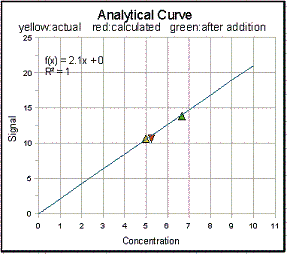You can see the analytical curve changes slope as you do this, but that both the sample signal (yellow triangle) and the standard signal (green triangle) track this change, and so the calculated sample concentration (red triangle) remains accurate.

Now try setting blank to 1 or 2, to test the affect of an additive interference. Unfortunately, the standard addition method does not correct for additive interferences, only for multiplicative interferences. (You have to rely on other methods to compensate for additive interferences, such as multiwavelength methods, wavelength modulation, derivative methods, peak fitting, high-resolution spectroscopy, separation methods, etc). Also, a linear analytical curve is a requirement.

Set Ev and Es=1 to introduce a small random error. The predicted standard deviation of Cx (Cell C70) is computed by breaking down the equation for Cx into a series of differences, sums, products, and ratios, and applying the rules for error propagation to each step. These error propagation calculations are performed in cells D111:F118.  Comparing the measured RSD of this method with that of the single standard method, you can see that the single standard addition method is less precise by about a factor of 2, which might seem surprising considering that both methods measure the unknown sample along with a single standard solution. You can understand what is going on here by looking at the expressions for Cx for the two methods: for the single standard method, it is Cx=Cs*Sx/Ss; for the standard addition method, it is Cx = Sx*Vss*Cs)/(Ss*(Vxx+Vss)-Sx*Vxx).  The extra volume terms Vss and Vxx, both of which are subject to random volumetric errors, do not occur in the single standard in the single standard method. Moreover, the denominator is the difference between two noisy quantities, Ss*(Vxx+Vss) and Sx*Vxx, which increases the relative standard deviation of the difference. The result is that the precision of standard addition is noticeably poorer than the single standard method, but this the price for correcting for multiplicative interference.

### The Multiple Standard Addition Method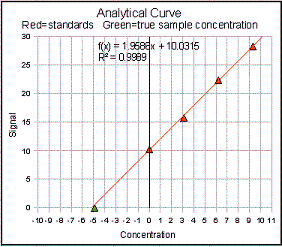The standard addition method can also be used with multiple standards: (StandardAdditionOO.ods , view Screen Shot). In this method a series of aliquots of the sample solution are taken, increasing amounts of standard material are added to each one, and the signals from the resulting mixtures are measured and plotted against the concentration of added standard. If the resulting calibration curve is sufficiently linear, a first-order least-squares fit of the data is computed. The sample concentration is given by the negative of the x-axis intercept (and to the ratio of the y-intercept to the slope). The advantage over the single addition method is that you can verify the linearity of the calibration curve.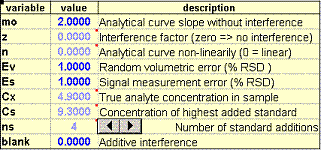To test this method, keep the same conditions as before (mo=2, blank=0, Ev and Es=0, z=0, n=0, Cx=5, and Cs=10) and set the number of standards ("ns") to 4. You can see that the calibration curve is linear and that the x-axis intercept is exactly -5 (which agrees with the negative of Cx). Now increase the interference (variable "z") to introduce an increasingly severe multiplicative interference. You can see the analytical curve changes slope as you do this, but that the x-axis intercept remains unchanged, proving that this method corrects perfectly for multiplicative interferences (slope changes).  If change the analyte concentration Cx, the whole curve slides up and down, so that the x-axis intercept tracks the changes in Cx.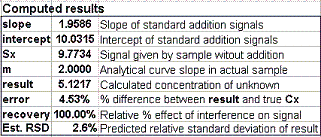Now introduce some random error:  set
Ev and Es = 1%. The calibration curve still looks pretty good, but as you change the interference ("z") or press f9 to recalculate, the  x-axis intercept changes slightly, as does the "result" in cell C65. The predicted relative standard deviation of Cx (cell C68) is the quadratic sum (square root of the sum of the squares) of the relative standard deviations of the slope and intercept computed by the curve fitting procedure. These error propagation calculations are performed in cells B82:F87. Note that the %RSD of 20 repeats (cell C72) is about 2.6%, significantly greater than Ev or Es, and is only roughly predicted by the Est. RSD (cell C68). However, if you increase the number of standards ("ns") to 16, the %RSD of 20 repeats is about half that with ns=4 and is much better predicted by the Est. RSD (both about 1.3%).  As you saw before, in the linear calibration curve method, the predicted RSD (because it is based on a single calibration curve) is extremely unreliable when the number of standards is small, and secondly, the %RSD of the result improves slightly when the number of standards is increased greatly.

If you compare the precision of this method to that of the linear calibration curve method, you'll notice that the multiple standard method is poorer, even though their expressions for Cx are very similar: Cx = (Sx-intercept)/slope v. Cx = intercept/slope.  Here again, correlation between terms is significant: there is sufficient negative correlation between the intercept and the slope in the multiple standard method (the intercept goes down when the slope goes up and vice versa) that the relative standard deviation (RSD) of the ratio of the two is poorer than the square root of the sum of the squares of the relative standard deviations of the two terms individually (as would be the case if they were not correlated).

### The Bottom Line

The take-home lesson here is two-fold:
1. Each calibration method has its own advantages and disadvantages, compared in Table 1 below; there is not one method that is best in all aspects and none that compensate or eliminate all possible errors (none, for example, eliminate additive interferences). As expected, the simplest methods do the least; the more complex methods do more, but at a cost.
2. The random error (relative standard deviation) of the measured concentrations (Table 2) is typically poorer (greater) than that of the volumetric precision or the signal precision alone, depending on the calibration method, but is usually no more than twice (except for the single-addition method).

Table 1: Comparison of calibration methods
Calibration Method
................................................
Complexity
(1=low; 5=high)
Detect non-linearity? Correct non-linearity? Correct multiplicative interference? Correct additive interference?
Single standard 1
 no
no no no
Bracket 2 yes partial no no
Calibration Curve, linear 3 yes no no no
Calibration Curve, non-linear 4 yes yes no no
Single standard addition 2 no no yes no
Multiple standard addition 5 yes no yes no

Table 2: Precision of calibration methods

(conditions: Cx = 10, highest standard = 10, Ev = Es = 1%, zero non-linearity, zero interferences)

 Method ................................................. Volumetric error Signal error Predicted RSD Measured RSD Single standard 1% 1% 1.7% 1.7% Bracket 1% 1% 2.5% 1.8% Calibration Curve linear 1% 1% 1.4% 1.4% (10 standards) Calibration. Curve Quadratic 1% 1% -- 2.0% (10 standards) Single standard addition 1% 1% 3.4% 3.4% Multiple standard addition 1% 1% 2.5% 2.5% (10 standards)

Table 3: Effect of number of standards for Linear Calibration Curve
(Same conditions as above)

 Number of standards RSD of slope SD of intercept Predicted RSD Measured RSD 4 1.4 0.1 2 2 16 0.7 0.05 1.7 1.7

Student assignment for Standard Addition Method, WingZ version:

#### The Single Standard Addition Method (Old 1992 version)

Our textbook, Ingle and Crouch, Chapter 6, page 179, says "The standard addition procedure is a powerful technique that is often used improperly due to a failure to understand the assumptions involved."  This simulation will help you appreciate the capabilities and limitations of the standard addition procedure.

1. Open StandardAddition.wkz. This model is based on the text, page 178-179 and Equation 6-16. The same terminology is used, with the following modifications: Ss is used for the signal measured after standard addition instead of Sx+s. Cx means the true analyte concentration (the unknown in the simulated experiment); the experimental quantity calculated by equation Equation 6-16, which is supposed to be a measure of Cx, is called "result". The volumes Vx and Vs mean the actual volumes (including the random volumetric errors); nomVx and nomVs are the "nominal" volumes, that is, the labeled volumes of the pipettes and flasks.

2. The simulation includes the effect of a multiplicative interference (Io = interferent concentration) and additive interference, i.e. blank error (blank = uncorrected blank signal), and random errors in volume and signal measurement. Errors are assumed to be a fixed percentage of the quantity measured (fixed relative error rather than fixed absolute error). The analytical curve is assumed to be linear.

3. The following are the independent variable that you can change:

 mo Analytical curve slope without interference z Interference factor (zero => no interference) Io Interferent concentration in original sample Ev Random volumetric error (% RSD ) Es Signal measurement error (% RSD) Cx Analyte concentration in original sample solution Cs Analyte concentration of standard solution blank (Uncorrected) blank signal nomVx Nominal volume of sample solution before addition nomVs Nominal volume of standard added to sample

To change any of these, click on the number (not on the symbol) in the spreadsheet, type a new value, and press the enter key. The other quantities in the spreadsheet are dependent variables that are calculated from these independent variables. The most important of these is result, which is the experimental estimate of Cx calculated by equation Equation 6-16. In this simulation we will compare result to the correct value Cx to see how well Equation 6-16 works.

4. Choose any value of Cx and nomVx you like, then set Cs = ten-fold or so larger than Cx. Start with the ideal case of no interference  (Io = 0; blank = 0) and no random errors (Ev = 0 and Es = 0). Verify that result = Cx for arbitrary Cs, nomVx, and nomVs.

5. Introduce a multiplicative interference by making Io > 0 and z > 0, keeping blank = 0. (The recovery expresses by what percent the analytical signal is changed by the interference). Does result = Cx? Try arbitrary values of Io, z, Cx, Cs, nomVx, and nomVs and notice the effect on result.

6. Introduce an additive interference by making blank > 0. Compare result and Cx. What do you conclude about the ability of the standard addition method to compensate for additive and multiplicative interferences?

7. Introduce random errors into the volumetric measurement (Ev) and the signal measurement (Es). To start with make both 1% RSD (Ev = Es =1). Set Io > 0 and z > 0, keeping blank = 0 to simulate a multiplicative interference only. Click on the 20 repeat runs button to simulate 20 separate standard addition measurements. (Quick repeat does the same thing, only faster). The table on the right shows the result of each measurement, and at the bottom computes the mean, standard deviation (s), percent relative standard deviation, and the error (% difference between the mean and Cx). Why is it that if you perform several successive 20-run simulations under fixed conditions, the standard deviation is the exactly the same each time? How could the simulation be designed to make the standard deviation more reproducible?

8. Vary Cs and nomVs and observe the effect on the percent relative standard deviation of the 20 repeats. Is there an optimum value of Cs and nomVs that minimizes this error? On the basis of your observations, formulate a rule that allows you to predict the optimum value of Cs and nomVs.

9. Why is it that, even under the best condition, the % RSD of result is greater than Es or Ev?

(c) T.C. O'Haver, 1992 (WingZ versions), 2009 (OpenOffice versions). (c) 1991, 2015. This page is part of Interactive Computer Models for Analytical Chemistry Instruction, created and maintained by Prof. Tom O'Haver , Professor Emeritus, The University of Maryland at College Park. Comments, suggestions and questions should be directed to Prof. O'Haver at toh@umd.edu. Last updated August, 2014.
Number of unique visits since May 17, 2008: